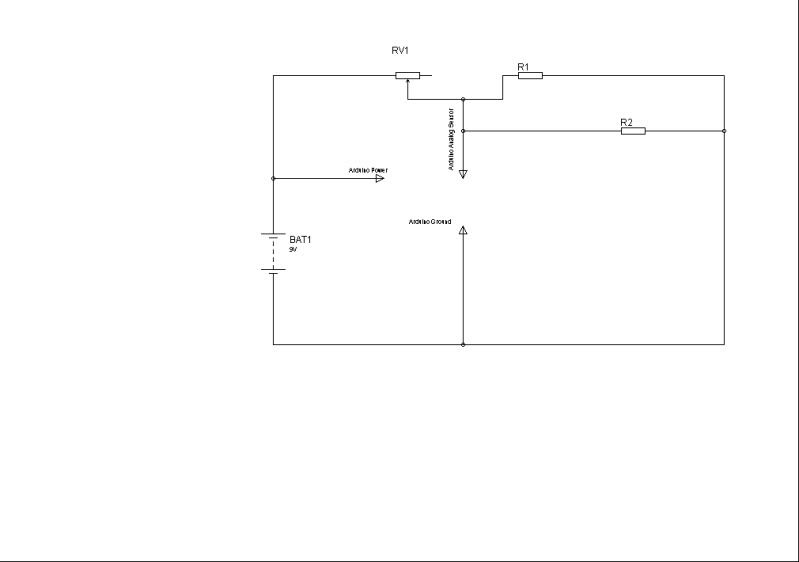Hi Everyone, I am having some problems reading an analog input 0-5v from a motorcycle gear position sensor with my arduino. when i check the voltage going into the analog pin on the arduino (with a voltmeter) it reads the correct value, 0 to 4.6v depending on the gear of the engine. however, the arduino is always reading 0 from the analog pin and i can't figure out why that is. any help would be very much appreciated.

Here is a wiring diagram that shows how i have it hooked up. The two resistors at the top of the image represent the Gear position sensor.

Thank you.Check the continuity on the ground. Specifically when you measure the voltage going in to the arduino you put one probe on the arduino input, where do you put the other? Check that there is a path between the place of the other voltage probe and the arduino ground.

Then post the sketch because it might be that you are doing something wrong in software.

When checking the voltage we are checking it by intercepting the wire going into analog pin 5 (our analog input) and the ground from the voltmeter is going directly to battery ground. the path is continuous from the arduino ground to the battery ground.

to check the analog input we are just using the smoothing code from the example included with the ardunio ide. but i will post it anyway.

int index = 0;                  // the index of the current reading
int total = 0;                  // the running total
int average = 0;                // the average

int inputPin = 5;

void setup()
{
// initialize serial communication with computer:
Serial.begin(9600);
// initialize all the readings to 0:
}

void loop() {
// advance to the next position in the array:
index = index + 1;

// if we're at the end of the array...
// ...wrap around to the beginning:
index = 0;

// calculate the average: